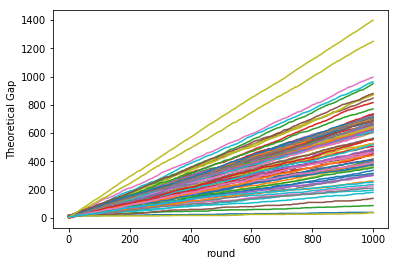In this post, we demonstrate the theoretical gurantee on the performance of the Weighted Majority Alogorithm (WMA) with empirical validation. WMA is one of the beautiful algorithms in the framework of online learning and sequential decision making under uncertainity.

First, let’s describe a setup where WMA can be used. Assume that a friend of yours challenges to a True or False quiz where you’re allowed to seek help / advice from $n$ advisors throughout the game.

With this challenge at hand, WMA helps you play the game such that the number of your mistakes is upper bounded by roughly twice the number of mistakes made by your best advisor, i.e., the least number of mistakes made among your $n$ advisors. In the next paragraph, we’ll see how you can use WMA in the game.

For each advisor $i$ and question (round) $t$, WMA associates a weight $w^t_i$. With $w^1_i=1\;, \forall i=1,\ldots, n.$ Assume the game has $T$ rounds. If advisor $i$ makes a mistake at round $t$, its weight is adjusted by a multiplicative factor $1-\eta$ where $\eta\in(0,1/2)$. That is, $w^{t+1}_i= (1-\eta) w^t_i$ if $i$ makes a mistake. Otherwise, $w^{t+1}_i=w^t_i$. At each round $t$, your decision is governed by the sum of weights of advisors who think the answer is True versus that of those who think the answer should be False. Mathematically, denote your answer at round $t$ by $y^t$, and the advisors’ answers by $x^t_i$. These variables take the values $0$ and $1$ to represent True and False respectively: we have

Let’s put WMA in action. Below, I have created $n$ advisors whose decisions at each round are based on tossing coins whose bias is sampled uniformly, $p_i\sim \mathcal{U}(0,1)$. Likewise, the game generates questions whose answers follows a Bernoulli distribution with $p_g\sim\mathcal{U}(0,1)$. We denote the correct answer at round $t$ by $z^t$. Also, let’s try to validate the theoretical bound on its performance. From this, after $\hat{t}$ rounds,

That is,

Let’s verify the above with the following 100 games with 1000 rounds each.

import numpy as np

# let's try 100 games each with 1000 rounds,
num_runs = 100
T = 1000

diff = np.zeros((num_runs, T))
for run in range(num_runs):
n = np.random.randint(1,10)
ps = np.random.random(n)
ws = np.ones(n)
p_g = np.random.random()
eta = 0.25

m = 0
ms = np.zeros(n, dtype=np.int32)

for t in range(T):
z = np.random.random() >= p_g
xs = np.random.random(n) >= ps
y = np.sum(xs * ws) >= np.sum((1-xs) * ws)

m += (y != z)
ms[xs != z] += 1

# theoretical bound
th_ub = 2 * (1+eta) * min(ms) + 2. * np.log(n) / eta
diff[run, t] = th_ub - m

# update weights
ws[xs != z] = (1-eta) * ws[xs != z]

assert np.min(diff) >= 0, "Theoretical gap should be non-negative"


Our mistakes never exceeded the theoretical upper limit, as can be seen from the non-negative theoretical gap across all the runs and rounds of the game.

import matplotlib.pyplot as plt

_ = plt.plot(diff.T)
plt.xlabel('round')
plt.ylabel('Theoretical Gap')
plt.show()